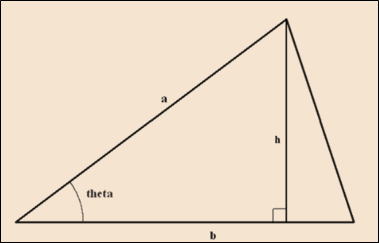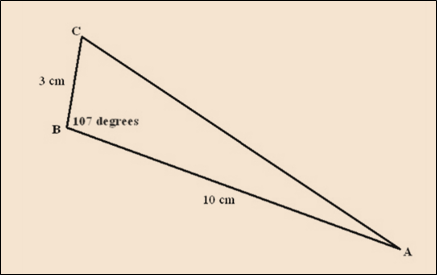# A = 1 2 a b sin θ### Single Variable Calculus: Concepts...

4th Edition
James Stewart
Publisher: Cengage Learning
ISBN: 9781337687805### Single Variable Calculus: Concepts...

4th Edition
James Stewart
Publisher: Cengage Learning
ISBN: 9781337687805

#### Solutions

Chapter C, Problem 46E

(a)

To determine

## To show:   A=12absinθ

Expert Solution

### Explanation of Solution

Given information:

Length of two sides: a,b

Angle: θ

Formula Used:

The area of triangle is given by the following expression:

A=12bh

Where,

Base: b

Height: h

Proof:

First sketch the triangle with two side length as a,b and includes an angle of θ . Then height h as perpendicular to the side b :From figure:

sinθ=hah=asinθ

So, the area of triangle is given by the following expression:

A=12bhA=12b(asinθ)A=12absinθ

Hence, proved.

(b)

To determine

### To calculate: The area of ΔABC

Expert Solution

The area of ΔABC is 14.34457cm2 .

### Explanation of Solution

Given information:

|AB|=10cm|BC|=3cmABC=107°

Formula Used:

The area of triangle is given by the following expression:

A=12bh

Where,

Base: b

Height: h

Calculation:

ΔABC is drawn as follow with the given information:The area of triangle is given by the following expression:

A=12bh

Put the given data:

A=12bhA=12|AB||BC|ABCA=12(10×3×107)A=14.34457cm2

### Have a homework question?

Subscribe to bartleby learn! Ask subject matter experts 30 homework questions each month. Plus, you’ll have access to millions of step-by-step textbook answers!